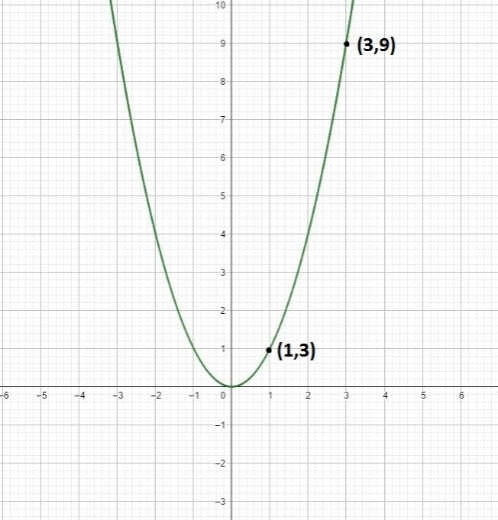Chapter 1, Problem 1RE

Chapter
Section
Textbook Problem

Precalculus or Calculus In Exercises 1 and 2, decide whether the problem can be solved using precalculus or whether calculus is required. If the problem can be solved using precalculus, solve it. If the problem seems to require calculus, explain your reasoning and use a graphical or numerical approach to estimate the solution.Find the distance between the points (1, 1) and (3, 9) along the curve y = x 2 .

To determine

To calculate: distance between the points (1,1) and (3,9) along the curve y=x2.

Solution: The distance between the points (1,1) and (3,9) along the curve y=x2 is 8.26815.

Explanation

Given:

The curve y=x2 and the points (1,1) and (3,9)

Calculation:

To the solve this problem we need use calculus as the path between the points, (1,1) and (3,9) is nonlinear.Applying the formula, L(x1,x2)=x1x21+[f(x)]2dx, where L(x1,x2) is the pathbetween the points (1,1) and (3,9) along the curve y=x2.

Here, f(x)=x2 and f(x)=2x.

Thus, L(x1,x2)=131+4x2dx8.26815

The Solution to Your Study Problems

Bartleby provides explanations to thousands of textbook problems written by our experts, many with advanced degrees!

Get Started

A sample with a mean of M = 8 has X = 56. How many scores are in the sample?

Essentials of Statistics for The Behavioral Sciences (MindTap Course List)

2. Define the terms population, sample, parameter and statistic.

Statistics for The Behavioral Sciences (MindTap Course List)

Find all the answers to your study problems with bartleby.
Textbook solutions plus Q&A. Get As ASAP arrow_forward